Writing Equation of Line

Write the equation of the line passing through the
points A(2,-5) and B(4,1).

Solution:
 Normal Solution:  Of course you can simply use your calculator to help you find the slope (using the slope formula) and substitute your values into the point-slope form for the equation of a straight line. Slope Formula: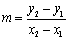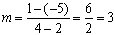Point-Slope Form for Line: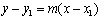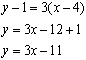Calculator Solution:  The Statistics features of the graphing calculator can be used to write the equations of straight lines given points. 1.  Enter the points into lists L1 and L2.        (See Basic Commands for entering data.) Be careful to keep the x and corresponding y coordinates lined up horizontally.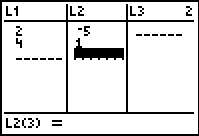2.   Choose STAT.  Arrow to the right to CALC at the top of the screen.  Choose #4:LinReg(ax+b).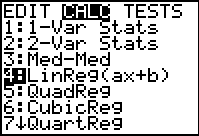3.   On the home screen you will need to tell the calculator which lists contain your points.  If you also wish to quickly graph your new line, include Y1.(L1 and L2 are above the numbers 1 and 2 on the calculator.)(To get Y1:  Choose VARS → Y-VARS, #1Function.  Choose Y1)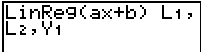The Y1 is only needed if you wish  to also see the graph of the line. 4.  Hitting ENTER will show the screen at the right.  "a" is the slope, and "b" is the y-intercept. If you hit y = , you will see the equation for the line.  Hitting GRAPH will show the graph.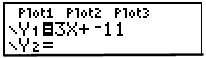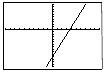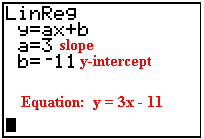Finding Your Way Around TABLE of  CONTENTS